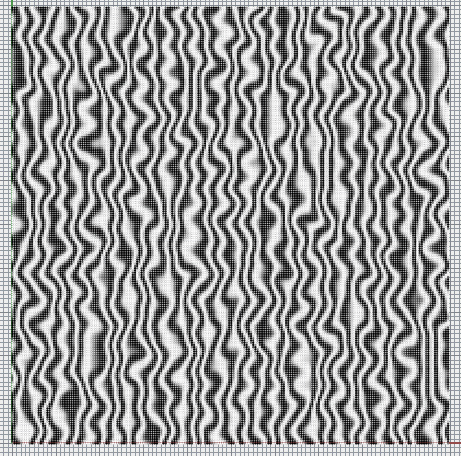# Write a Bitmap Pixel by Pixel in Python?

looked in to System.Drawing import Bitmap, Also I am aware of Pillow but not sure how to install on Rhino Iron Python
basically need to save the image below which is now a list of color valuesThis is hardly the most efficient method, but probably the most simple (using .NET directly and in this case for reading an Excel/CSV file and converting that to a bitmap):

``````import System.Drawing as sd

csvData = []
l = line.strip().split(';')
csvData.append(l)

if Write and csvData:

# Get number of columns and rows in excel data
columns = len(csvData)
rows = len(csvData)

# Make bitmap
bm = sd.Bitmap(columns,rows)

for i in range(columns):
for j in range(rows):

# Get data in cell
cd = csvData[j][i]

# Make color depending on cell data
if cd in ["a","A"]:
col = sd.Color.White
elif cd in ["y","Y"]:
col = sd.Color.Black
elif cd in ["B"]:
col = sd.Color.Yellow
elif cd in ["W"]:
col = sd.Color.Gray

# Set pixel
bm.SetPixel(i,j,col)

# Save to file
bm.Save(PathWrite,sd.Imaging.ImageFormat.Bmp)
``````

160104_CSVToBitmap_00.gh (4.9 KB)

3 Likes

Here is my take just by looping through a list of colors to write, The down side is it takes 30 mins to do 600px image vs C# less than a minute

``````import System.Drawing
import math

bmp = System.Drawing.Bitmap(Width,Height)

for x in xrange(Height*Width):
#index = i + j*Width
j = int(math.floor(x/Width))
i = int(math.floor(x-(j*Width)))
col = Color[x]
bmp.SetPixel(i,j,col)
bmp.Save(PathWrite,System.Drawing.Imaging.ImageFormat.Bmp)``````

Wow that’s slow! Can you time the bmp creation part and writing part separately to see where ir’s Taking so long?
You should be able to use

``````j= x // Width
i= x % Width
``````

To simplify the code
Or even `j, i = divmod(x, Width)` which does the same

Ahh spotted it - you are saving the bitmap 600 times! No wonder it’s slowGot it Thanks!! 900ms

``````import System.Drawing as sd
import math

bm = sd.Bitmap(Width,Height)

for x in xrange(Height*Width):
j= x // Width
i= x % Width
col = Color[x]
bm.SetPixel(i,j,col)
bm.Save(PathWrite,sd.Imaging.ImageFormat.Bmp)``````

Not 600 times but likely more times: widthxheight. For a full HD that is 1920x1080 = 2 073 600 times (:

You’re saving the bitmap to file within the loop, which will be super duper slow. If moving the bitmap save outside of the loop is still too slow, consider using the more advanced `lockbits` method (as discussed here).

Edit: Looks like you guys already found the culprit. As you were.

Is there a way to show the image after it is saved, other than going to the file destination and opening it?

I usually `import os` and use `os.startfile(path)` for opening files upon creation.

1 Like

Nice, this beats fiddling with those awful forms…1 Like